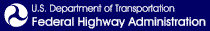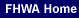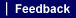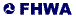Orders > Correspondence Manual > Appendix F

APPENDIX F. NUMBERS

Word Version - A viewer is available from Microsoft® for Word files.

Whether to express a number in figures or in words is often a troublesome choice. Here are some general guidelines in making that choice. Also, see the latest GPO Style Manual.

1. Use figures for 10 and for all numbers above 10.

 10 children 140 children
2. Units of measurement and time, actual or implied, are expressed in figures even when the number is 10 or below.

 6 years old 3 pints 4:30 p.m 7 days. June 2003 2 inches
3. Use figures to express sums of money.

 \$4.95 \$6 35 cents
4. When a sentence has two or more related numbers and one number is 10 or over, use figures for all the numbers.

Each of 15 major commodities (9 metal and 6 nonmetal) was in supply.

Each of nine major commodities (five metal and four nonmetal) was in supply.

5. Use figures to express most decimal fractions. If the value is less than one, place a zero before decimal point.

6. Fractions standing alone are generally spelled out (three-fourths of an inch). Mixed fractions are always expressed in figures (3 ½ cans). Fractions used as a unit modifier 1/2 –inch pipe are expressed in figures.

7. Use figures to indicate definite percentages.

 3 percent 5.5 percent 22 percent
8. Use figures to precede the words million and billion when stating a specific quantity of something.

 \$3 billion debt 24 million people
9. In phrases where consecutive numbers might be confusing, the shortest number is written as a word and the longest as a figure.

 9 two-story houses two 7-month contracts
10. Use the same form for all numbers that occur in the same context.

 5 sheep, 3 horses, 45 pigs, and 101 goats
11. Use words for all numbers that are indefinite expressions.

 He has been with the company since the early fifties.
12. Use figures to indicate degrees of latitude and longitude and to indicate temperature.

 45ºS 98.6º
13. Use figures to indicate time of day when followed by either p.m. or a.m.

 5 a.m. 4:30 p.m.
14. For emphasis, when the time of day is followed by o'clock use figures. In formal correspondence, such as when writing to the White House, Members of Congress, Ambassadors, etc. use words.

 3 o'clock (emphasis) three o'clock (formal) Do not use "a.m. or p.m." together with "o'clock."